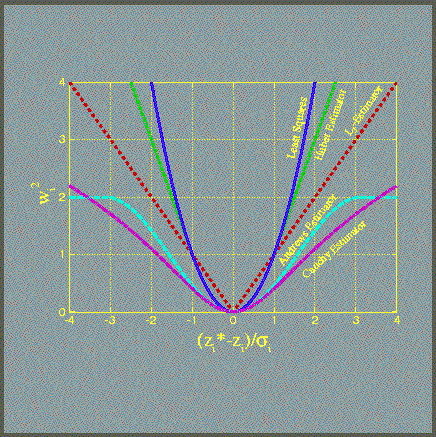# Objective Function

The objective function measures the misfit between the data and the corresponding modeling result. The standard method is weighted least squares, i.e., the objective function to be minimized is the sum of the residuals weighted by the inverse of the measurement error. The objective function can be derived from maximum likelihood considerations. For example, least-squares assumes that the measurement errors follow a normal distribution. However, if the residuals are not normally distributed, a different objective function should be chosen, or the parameters are not maximum-likelihood estimates. As an alternative to least-squares, iTOUGH2 provides a number of additional functions. These so-called robust estimators assign less weight to large residuals, potentially reducing the impact of outliers and systematic errors on the parameter estimates. The robust estimators are described in Finsterle and Najita .Here is a list of the objective functions available in iTOUGH2:

• Least-squares
• L1-estimator
• Huber estimator
• Cauchy estimator
• Andrews estimator